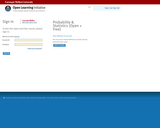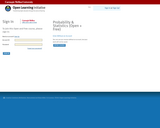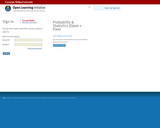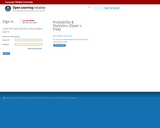Updating search results...

# 6 Results

View
Selected filters:
• Carnegie Mellon UniversityConditional Remix & Share Permitted
CC BY-NC-SA
Rating
0.0 stars

Apply the sampling distribution of the sample mean as summarized by the Central Limit Theorem (when appropriate). In particular, be able to identify unusual samples from a given population.

Subject:
Mathematics
Statistics and Probability
Material Type:
Module
Provider:
Carnegie Mellon University
Provider Set:
Open Learning Initiative
02/28/2018Conditional Remix & Share Permitted
CC BY-NC-SA
Rating
0.0 stars

1). Summarize and describe the distribution of a categorical variable in context.
2). Generate and interpret several different graphical displays of the distribution of a quantitative variable (histogram, stemplot, boxplot).
3). Summarize and describe the distribution of a quantitative variable in context: a) describe the overall pattern, b) describe striking deviations from the pattern.
4). Relate measures of center and spread to the shape of the distribution, and choose the appropriate measures in different contexts.
5). Compare and contrast distributions (of quantitative data) from two or more groups, and produce a brief summary, interpreting your findings in context.
5). Apply the standard deviation rule to the special case of distributions having the "normal" shape.

Subject:
Mathematics
Statistics and Probability
Material Type:
Module
Provider:
Carnegie Mellon University
Provider Set:
Open Learning Initiative
02/28/2018Conditional Remix & Share Permitted
CC BY-NC-SA
Rating
0.0 stars

LEARNING OBJECTIVE: Identify and distinguish between a parameter and a statistic.

LEARNING OBJECTIVE: Explain the concepts of sampling variability and sampling distribution.

Subject:
Mathematics
Statistics and Probability
Material Type:
Module
Provider:
Carnegie Mellon University
Provider Set:
Open Learning Initiative
02/28/2018Conditional Remix & Share Permitted
CC BY-NC-SA
Rating
0.0 stars

Explain how a density function is used to find probabilities involving continuous random variables.

Subject:
Mathematics
Statistics and Probability
Material Type:
Module
Provider:
Carnegie Mellon University
Provider Set:
Open Learning Initiative
02/28/2018Conditional Remix & Share Permitted
CC BY-NC-SA
Rating
0.0 stars

Learning Objective: Apply probability rules in order to find the likelihood of an event.

Subject:
Mathematics
Statistics and Probability
Material Type:
Module
Provider:
Carnegie Mellon University
Provider Set:
Open Learning Initiative
02/28/2018Conditional Remix & Share Permitted
CC BY-NC-SA
Rating
0.0 stars

1). Identify the sampling method used in a study and discuss its implications and potential limitations.
2). Critically evaluate the reliability and validity of results published in mainstream media.
3). Summarize and describe the distribution of a categorical variable in context.

Subject:
Mathematics
Statistics and Probability
Material Type:
Module
Provider:
Carnegie Mellon University
Provider Set:
Open Learning Initiative